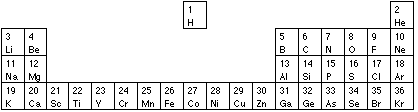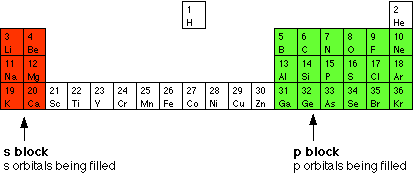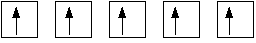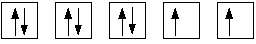Need help? Email help@shout.education

# Electronic Structures of Atoms

This page explores how you write electronic structures for atoms using s, p, and d notation. It assumes that you know about simple atomic orbitals – at least as far as the way they are named, and their relative energies. If you want to look at the electronic structures of simple monatomic ions (such as Cl-, Ca2+ and Cr3+), you will find a link at the bottom of the page.

## The Electronic Structures of Atoms

### Relating Orbital Filling to the Periodic TableNote: On some screens the V for vanadium (element 23) may look a bit like a Y. This isn't a mistake, but an effect of converting my original diagram into a lower quality gif image for efficient web use.

UK syllabuses for 16 – 18 year olds tend to stop at krypton when it comes to writing electronic structures, but it is possible that you could be asked for structures for elements up as far as barium. After barium you have to worry about f orbitals as well as s, p and d orbitals – and that's a problem for chemistry at a higher level. It is important that you look through past exam papers as well as your syllabus so that you can judge how hard the questions are likely to get.

This page looks in detail at the elements in the shortened version of the Periodic Table above, and then shows how you could work out the structures of some bigger atoms.

Important! You must have a copy of your syllabus and copies of recent exam papers. If you are studying a UK-based syllabus and haven't got them, follow this link to find out how to get hold of them.

#### The first period

Hydrogen has its only electron in the 1s orbital – 1s1, and at helium the first level is completely full – 1s2.

#### The second period

Now we need to start filling the second level, and hence start the second period. Lithium's electron goes into the 2s orbital because that has a lower energy than the 2p orbitals. Lithium has an electronic structure of 1s22s1. Beryllium adds a second electron to this same level – 1s22s2.

Now the 2p levels start to fill. These levels all have the same energy, and so the electrons go in singly at first.

 B 1s^22s^2\color{#467abf}{2p_x^1} C 1s^22s^22p_x^1\color{#467abf}{2p_y^1} N 1s^22s^22p_x^12p_y^1\color{#467abf}{2p_z^1}

Note: The orbitals where something new is happening are shown in blue. You wouldn't normally write them any differently from the other orbitals.

The next electrons to go in will have to pair up with those already there.

 O 1s^22s^2\color{#467abf}{2p_x^2}2p_y^12p_z^1 F 1s^22s^22p_x^2\color{#467abf}{2p_y^2}2p_z^1 Ne 1s^22s^22p_x^22p_y^2\color{#467abf}{2p_z^2}

You can see that it is going to get progressively tedious to write the full electronic structures of atoms as the number of electrons increases. There are two ways around this, and you must be familiar with both.

Shortcut 1: All the various p electrons can be lumped together. For example, fluorine could be written as 1s22s22p5, and neon as 1s22s22p6.

This is what is normally done if the electrons are in an inner layer. If the electrons are in the bonding level (those on the outside of the atom), they are sometimes written in shorthand, sometimes in full. Don't worry about this. Be prepared to meet either version, but if you are asked for the electronic structure of something in an exam, write it out in full showing all the px, py and pz orbitals in the outer level separately.

For example, although we haven't yet met the electronic structure of chlorine, you could write it as 1s22s22p63s23px23py23pz1.

Notice that the 2p electrons are all lumped together whereas the 3p ones are shown in full. The logic is that the 3p electrons will be involved in bonding because they are on the outside of the atom, whereas the 2p electrons are buried deep in the atom and aren't really of any interest.

Shortcut 2: You can lump all the inner electrons together using, for example, the symbol [Ne]. In this context, [Ne] means the electronic structure of neon – in other words: 1s22s22px22py22pz2. You wouldn't do this with helium because it takes longer to write [He] than it does to write 1s2.

On this basis the structure of chlorine would be written [Ne]3s23px23py23pz1.

#### The third period

At neon, all the second level orbitals are full, and so after this we have to start the third period with sodium. The pattern of filling is now exactly the same as in the previous period, except that everything is now happening at the 3-level.

For example:

 electron configuration short version Mg 1s^22s^22p^6\color{#467abf}{3s^2} [Ne]3s^2 S 1s^22s^22p^63s^2\color{#467abf}{3p_x^23p_y^13p_z^1} [Ne]3s^23p_x^23p_y^13p_z^1 Ar 1s^22s^22p^63s^23p_x^2\color{#467abf}{3p_y^23p_z^2} [Ne]3s^23p_x^23p_y^23p_z^2

Note: Check that you can do these. Cover the text and then work out these structures for yourself. Then do all the rest of this period. When you've finished, check your answers against the corresponding elements from the previous period. Your answers should be the same except a level further out.

#### The beginning of the fourth period

At this point the 3-level orbitals aren't all full – the 3d levels haven't been used yet. But if you refer back to the energies of the orbitals, you will see that the next lowest energy orbital is the 4s – so that fills next.

 K 1s^22s^22p^63s^23p^6\color{#467abf}{4s^1} Ca 1s^22s^22p^63s^23p^6\color{#467abf}{4s^2}

There is strong evidence for this in the similarities in the chemistry of elements like sodium (1s^22s^22p^6\color{#467abf}{3s^1}) and potassium (1s^22s^22p^63s^23p^6\color{#467abf}{4s^1})

The outer electron governs their properties and that electron is in the same sort of orbital in both of the elements. That wouldn't be true if the outer electron in potassium was 3d^1.

#### s- and p-block elementsThe elements in Group 1 of the Periodic Table all have an outer electronic structure of ns^1 (where n is a number between 2 and 7). All Group 2 elements have an outer electronic structure of ns^2. Elements in Groups 1 and 2 are described as s-block elements.

Elements from Group 3 (the boron group) across to the noble gases all have their outer electrons in p orbitals. These are then described as p-block elements.

#### d-block elements

We are working out the electronic structures of the atoms using the Aufbau ("building up") Principle. So far we have got to calcium with a structure of 1s^22s^22p^63s^23p^64s^2.

The 4s level is now full, and the structures of the next atoms show electrons gradually filling up the 3d level. These are known as d-block elements.Once the 3d orbitals have filled up, the next electrons go into the 4p orbitals as you would expect.

d-block elements are elements in which the last electron to be added to the atom using the Aufbau Principle is in a d orbital.

The first series of these contains the elements from scandium to zinc, which at GCSE you probably called transition elements or transition metals. The terms "transition element" and "d-block element" don't quite have the same meaning, but it doesn't matter in the present context.

A transition element is one which has partially filled d orbitals either in the element or any of its compounds.

Zinc (at the right-hand end of the d-block) always has a completely full 3d level (3d^{10}) and so doesn't count as a transition element.

Some UK syllabuses use a more restrictive definition which defines a transition metal as one which has one or more stable ions with partly filled d orbitals. You don't need to worry about this until you do some transition metal chemistry.

d electrons are almost always described as, for example, d^5 or d^8 – and not written as separate orbitals. Remember that there are five d orbitals, and that the electrons will inhabit them singly as far as possible. Up to 5 electrons will occupy orbitals on their own. After that they will have to pair up.

d^5 meansd^8 meansNotice in what follows that all the 3-level orbitals are written together – with the 4s electrons written at the end of the electronic structure.

 Sc 1s^22s^22p^63s^23p^6\color{#467abf}{3d^14s^2} Ti 1s^22s^22p^63s^23p^6\color{#467abf}{3d^2}4s^2 V 1s^22s^22p^63s^23p^6\color{#467abf}{3d^3}4s^2 Cr 1s^22s^22p^63s^23p^6\color{#467abf}{3d^54s^1}

Whoops! Chromium breaks the sequence. In chromium, the electrons in the 3d and 4s orbitals rearrange so that there is one electron in each orbital. It would be convenient if the sequence was tidy – but it's not!

 Mn 1s^22s^22p^63s^23p^6\color{#467abf}{3d^54s^2} (back to being tidy again) Fe 1s^22s^22p^63s^23p^6\color{#467abf}{3d^6}4s^2 Co 1s^22s^22p^63s^23p^6\color{#467abf}{3d^7}4s^2 Ni 1s^22s^22p^63s^23p^6\color{#467abf}{3d^8}4s^2 Cu 1s^22s^22p^63s^23p^6\color{#467abf}{3d^{10}4s^1} (another awkward one!) Zn 1s^22s^22p^63s^23p^63d^{10}\color{#467abf}{4s^2}

And at zinc the process of filling the d orbitals is complete.

#### Filling the rest of period 4

The next orbitals to be used are the 4p, and these fill in exactly the same way as the 2p or 3p. We are back now with the p-block elements from gallium to krypton. Bromine, for example, is 1s^22s^22p^63s^23p^63d^34s^24p_x^24p_y^24p_z^1.

Useful exercise: Work out the electronic structures of all the elements from gallium to krypton. You can check your answers by comparing them with the elements directly above them in the Periodic Table. For example, gallium will have the same sort of arrangement of its outer level electrons as boron or aluminium – except that gallium's outer electrons will be in the 4-level.

### Summary

#### Writing the electronic structure of an element from hydrogen to krypton

• Use the Periodic Table to find the atomic number, and hence number of electrons.
• Fill up orbitals in the order 1s, 2s, 2p, 3s, 3p, 4s, 3d, 4p – until you run out of electrons. The 3d is the awkward one – remember that specially. Fill p and d orbitals singly as far as possible before pairing electrons up.
• Remember that chromium and copper have electronic structures which break the pattern in the first row of the d-block.

#### Writing the electronic structure of big s- or p-block elements

Note: We are deliberately excluding the d-block elements apart from the first row that we've already looked at in detail. The pattern of awkward structures isn't the same in the other rows. This is a problem for degree level.

First work out the number of outer electrons. This is quite likely all you will be asked to do anyway.

The number of outer electrons is the same as the group number. (The noble gases are a bit of a problem here, because they are normally called group 0 rather then group 8. Helium has 2 outer electrons; the rest have 8.) All elements in group 3, for example, have 3 electrons in their outer level. Fit these electrons into s and p orbitals as necessary. Which level orbitals? Count the periods in the Periodic Table (not forgetting the one with H and He in it).

Iodine is in group 7 and so has 7 outer electrons. It is in the fifth period and so its electrons will be in 5s and 5p orbitals. Iodine has the outer structure 5s^25p_x^25p_y^25p_z^1.

What about the inner electrons if you need to work them out as well? The 1, 2 and 3 levels will all be full, and so will the 4s, 4p and 4d. The 4f levels don't fill until after anything you will be asked about at A-level. Just forget about them! That gives the full structure: 1s^22s^22p^63s^23p^63d^34s^24p^64d^{10}5s^25p_x^25p_y^25p_z^1.

When you've finished, count all the electrons to make sure that they come to the same as the atomic number. Don't forget to make this check – it's easy to miss an orbital out when it gets this complicated.

Barium is in group 2 and so has 2 outer electrons. It is in the sixth period. Barium has the outer structure 6s2.

Including all the inner levels: 1s^22s^22p^63s^23p^63d^34s^24p^64d^{10}5s^25p^66s^2.

It would be easy to include 5d^{10} as well by mistake, but the d level always fills after the next s level – so 5d fills after 6s just as 3d fills after 4s. As long as you counted the number of electrons you could easily spot this mistake because you would have 10 too many.

Note: Don't worry too much about these complicated structures. You need to know how to work them out in principle, but your examiners are much more likely to ask you for something simple like sulfur or iron.